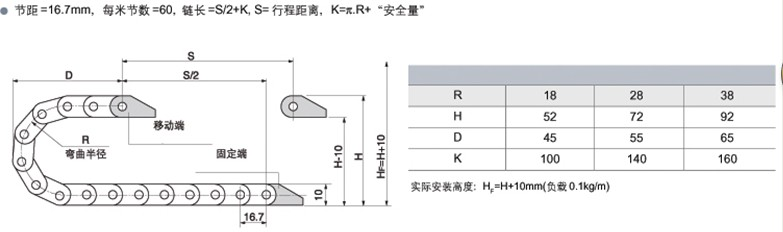R 15 28 H 25 72 D 37 55 K 52 140内高 内宽 外高 外宽 弯曲半径(R) 打开方式 对应接头孔距 7 7 10 12.5 15,28 B A=8

B为不可打开型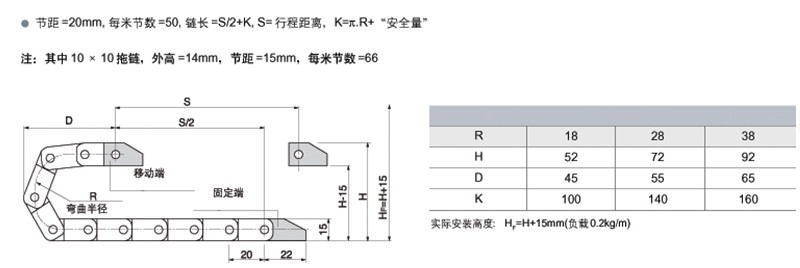内高 内宽 外高 外宽 弯曲半径（R) 打开方式 对应机头孔距 10 6 13.5 13.5 18 方便型 A=8 10 10 15 16 18,38 方便型 A=8 10 10 14 17.5 18,28 不可打开型 A=8 10 15 15.5 23 18,28,38 不可打开型 A=10 10 20 15.5 28.5 18,28 不可打开型 A=10 B=5

15系列：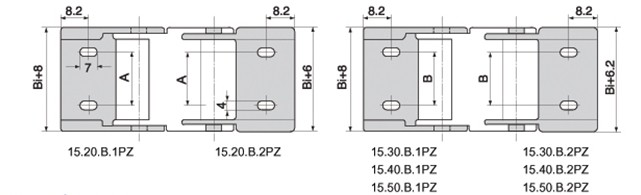内高 内宽 外高 外宽 弯曲半径（R) 打开方式 对应机头孔距 15 20 20 28.2 28,38,48 不可打开型 A=11 15 30 20 38.2 A=14 15 40 20 48.2 B=20 15 50 20 58.2 B=20

15系列-半封闭内侧可打开内高 内宽 外高 外宽 弯曲半径（R) 打开方式 对应机头孔距 15 15 20 23 28,38,48 半封闭内侧可打开 A=8 15 20 20 29 A=8 15 30 20 39 A1=22 B=9.2 15 40 20 49 A1-31.8 B=7.5 15 50 20 59 A1=41.8 B=7.5

18系列-半封闭内侧可打开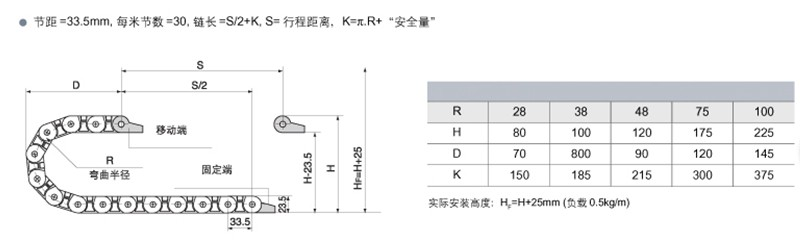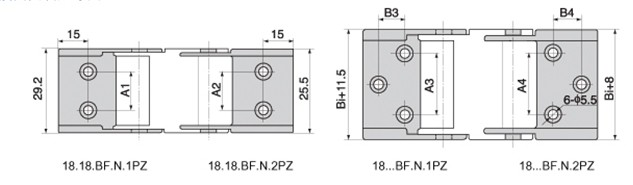内高 内宽 外高 外宽 弯曲半径（R) 打开方式 对应接头孔距 18 18 23.5 28 28,38,48,75,100 半封闭内侧可打开 A1=14，A2=9.5 18 25 23.5 35 A3=19.2，A4=13.6，B3=10，B4=10 18 37 23.5 47 A3=29，A4=25，B3=11，B4=11 18 50 23.5 60 A3=34.8，A4=34.8，B3=7.5，B4=7.5

18系列还有不可打开型和外侧打开型！

25系列-两测可打开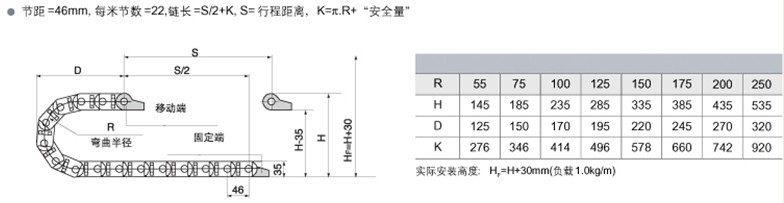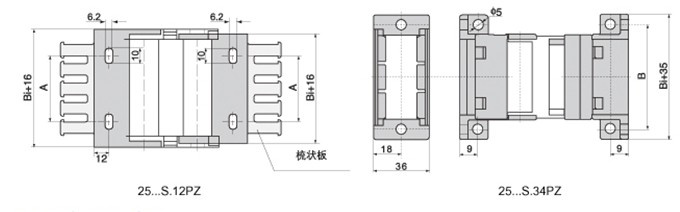内高 内宽 外高 外宽 弯曲半径（R) 打开方式 对应接头孔距 25 25 35 38 55，75，100，125，150，175，200，250 双侧可打开型 A=16 25 38 35 51 A=26 25 57 35 71 A=45 25 77 35 91 A=62